# The MDC Procedure

### Parameter Heterogeneity: Mixed Logit

One way of modeling unobserved heterogeneity across individuals in their sensitivity to observed exogenous variables is to use the mixed logit model with a random parameters or random coefficients specification. The probability of choosing alternative j is written as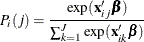whereis a vector of coefficients that varies across individuals and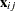is a vector of exogenous attributes.

For example, you can specify the distribution of the parameterto be the normal distribution.

The mixed logit model uses a Monte Carlo simulation method to estimate the probabilities of choice. There are two simulation methods available. If the RANDNUM=PSEUDO option is specified in the MODEL statement, pseudo-random numbers are generated; if the RANDNUM=HALTON option is specified, Halton quasi-random sequences are used. The default value is RANDNUM=HALTON.

You can estimate the model with normally distributed random coefficients of ttime with the following SAS statements:

/*-- mixed logit estimation --*/
proc mdc data=newdata type=mixedlogit;
model decision = ttime /
nchoice=3
mixed=(normalparm=ttime);
id pid;
run;


Let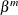and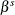be mean and scale parameters, respectively, for the random coefficient,. The relevant utility function is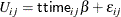where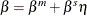(andare fixed mean and scale parameters, respectively). The stochastic component,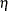, is assumed to be standard normal since the NORMALPARM= option is given. Alternatively, the UNIFORMPARM= or LOGNORMALPARM= option can be specified. The LOGNORMALPARM= option is useful when nonnegative parameters are being estimated. The NORMALPARM=, UNIFORMPARM=, and LOGNORMALPARM= variables must be included in the right-hand side of the MODEL statement. See the section Mixed Logit Model for more details. To estimate a mixed logit model by using the transportation mode choice data, the MDC procedure requires the MIXED= option for random components. Results of the mixed logit estimation are displayed in Figure 18.21.

Figure 18.21: Mixed Logit Model Parameter Estimates

The MDC Procedure

Mixed Multinomial Logit Estimates

Parameter Estimates
Parameter DF Estimate Standard
Error
t Value Approx
Pr > |t|
ttime_M 1 -0.5342 0.2184 -2.45 0.0144
ttime_S 1 0.2843 0.1911 1.49 0.1368

Note that the parameter ttime_M corresponds to the constant mean parameterand the parameter ttime_S corresponds to the constant scale parameter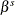of the random coefficient.